# Pivot Tables Review

In this article we'll give a quick introduction to Pivot Tables in Excel.

For example, let's say we had the following data in an Excel table: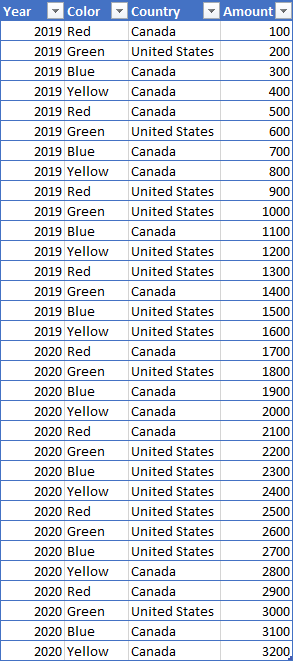If you were to create a pivot table with this data by dragging:

• Year into the Rows area
• Color into the Columns area
• Amount into the Values area: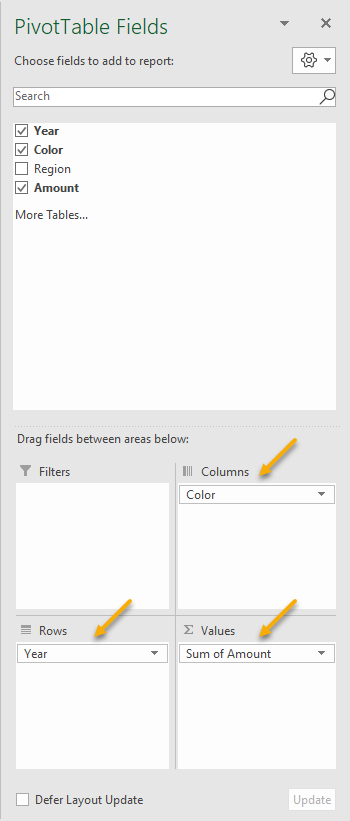You will see a pivot table with two rows, one for each year and four columns, one for each color: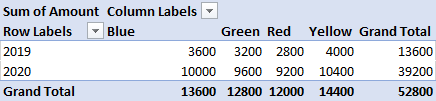Note that in a pivot table the only thing that can be represented in any given cell (for example the year 2019 and the color Green) is a number: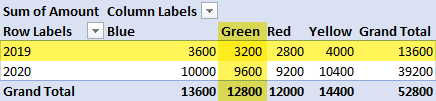Which, in this case, is the value 3200. That number is the sum total of the Amount column for all the rows that have the value 2019 for year and Green for color. Those rows can be viewed by filtering the original table to show only the appropriate data: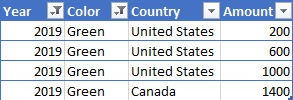That's all there really is to a Pivot table in Excel. To recap:

1. They are a way to easily group rows of data
2. Those groups can be created in combinations of Rows and/or Columns
3. For each intersection of Row and Column in the Pivot table, the source rows are filtered to only include the appropriate data
4. For the resulting rows one or more summary calculations are performed by dragging column names to Values
5. The output of the calculations are then visualized as cells in the Pivot table itself